# Ratios, Proportions, and Percents## Overview

Ratios, proportions, and percents cover a broad set of topics, and you need to be familiar with these topics because you will see several SAT math questions on these topics. In this section we’ll cover the following:

• Ratios and Rates
• Proportions
• Percents

Let’s look at each of these in more detail:

### Ratios and Rates

What is a ratio? It is a relationship between two quantities. Take a look at the definition.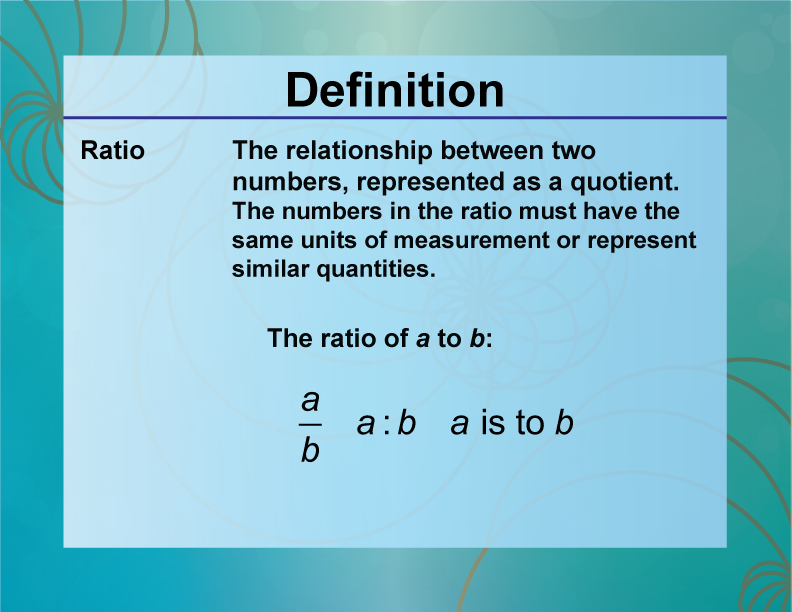Click on this link to learn more about ratios. This slide show includes a video and examples of different ratios. Here are some additional ratios topics you should review. Each of the following includes a link to a slide show with additional content:

A rate is a special type of ratio. Look at this definition.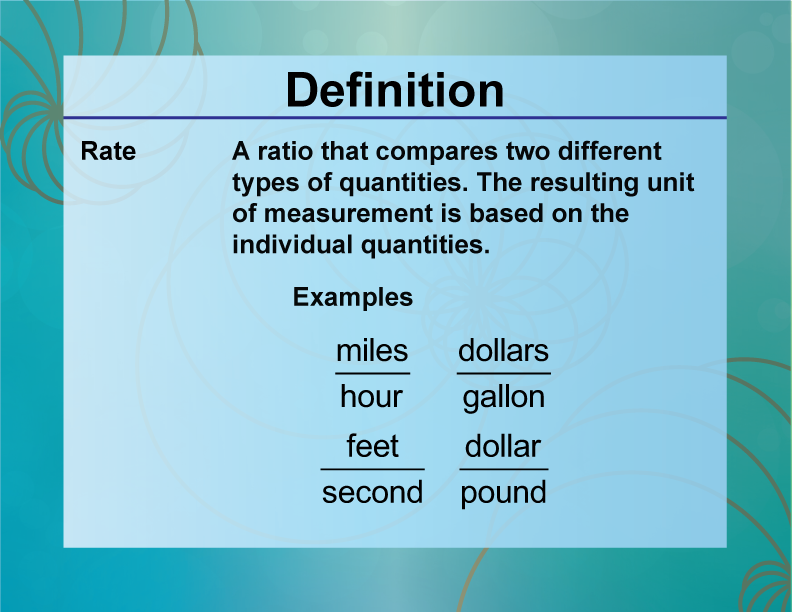Another topic related to rates is unit rates. Look at this definition.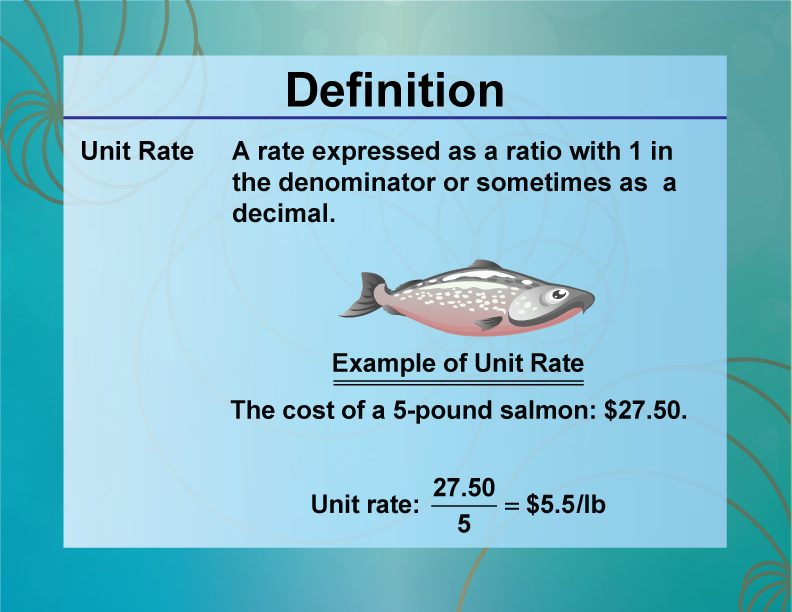SAT Skill: Ratios and Rates Example 1 A car moves 120 ft in 5 seconds. At that speed, about how many miles will it travel in 2 hours? This is a rate problem, so use the measurements to find the speed in ft/sec.Now convert to miles per hour:Now determine the distance traveled in 2 hours:Example 2 The Wifi at a hotel costs 60 cents a minute, after an initial cost of 4.99. Write an equation that can be used to find the total cost, c, in dollars per hour h. This is a rate problem. Take the cents per minute rate and convert it to a dollars per hour rate:Now you can write the equation for c:### Proportions

When two ratios are equal to each other, they can form an equation called a proportion. Look at this definition.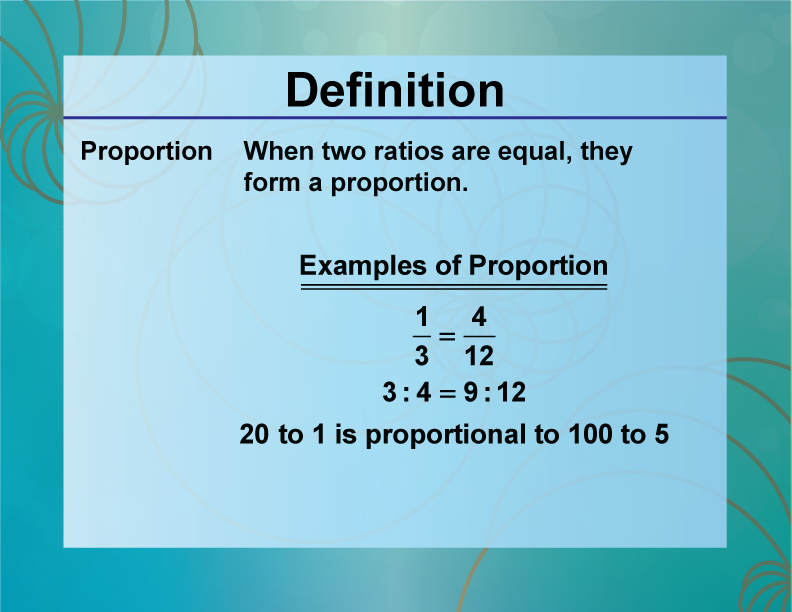When proportions include a variable, this results in an equation that can be solved.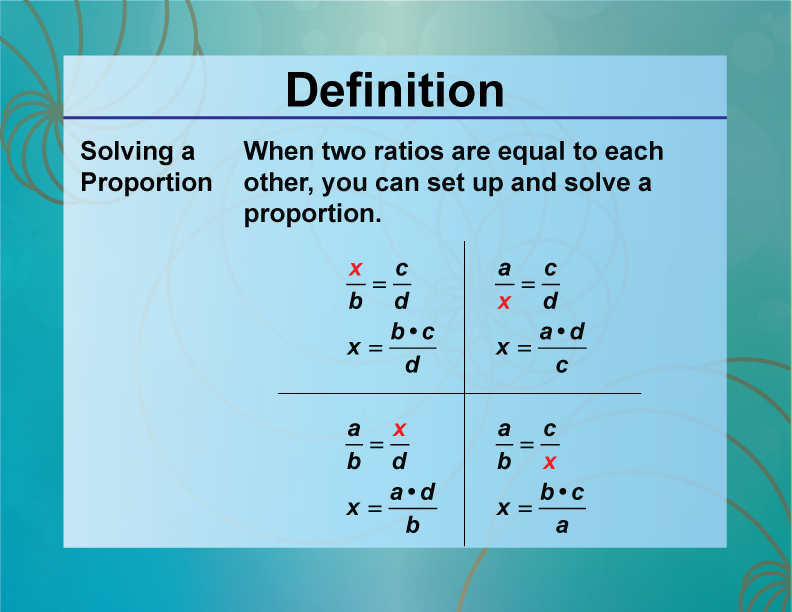SAT Skill: Proportions Example 1 A jar of peanut butter can be used to make two dozen sandwiches. How many jars of peanut butter are needed to make 240 sandwiches? This is a proportion problem. To make 240 sandwiches requires 480 slides of bread. Here are the equivalent ratios:Solve for x:### Percents

A percent is a ratio where the denominator is 100. You can also think of a percent as a fraction with a denominator of 100. Take a look at this definition.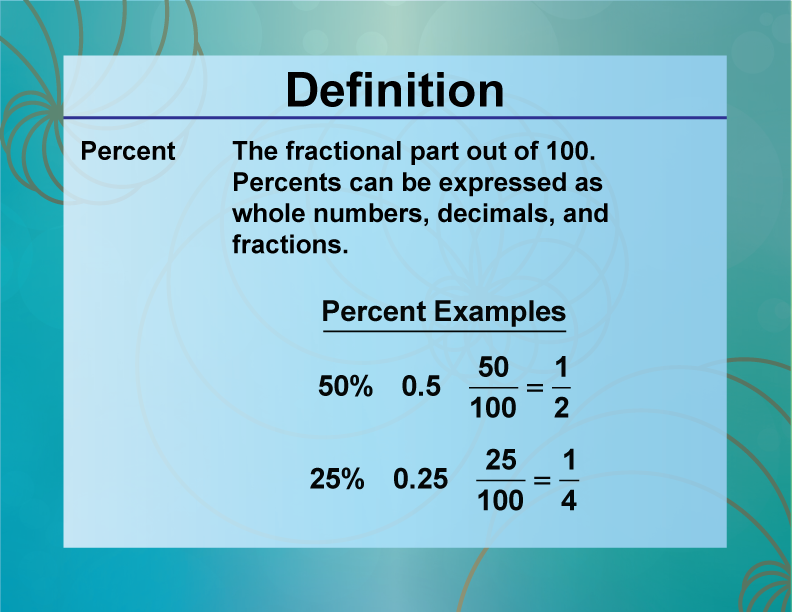To learn more about percents, click on this link. In working with percents, there are three different types of equations to solve. For each of the cases below, click on the link to see a slide show that provides additional content:

 SAT Skill: Percents Example 1 In a population of fish, 8% are tagged for tracking. If a total of 375 fish are released, how many of them are tagged? We are dealing with this case:Example 2 5 is 12% of what number? We are dealing with this case:Example 3 At a college 8% of the computer science majors were female. If there were 58 female computer science majors, what was the total number of computer science majors? We are dealing with this case: